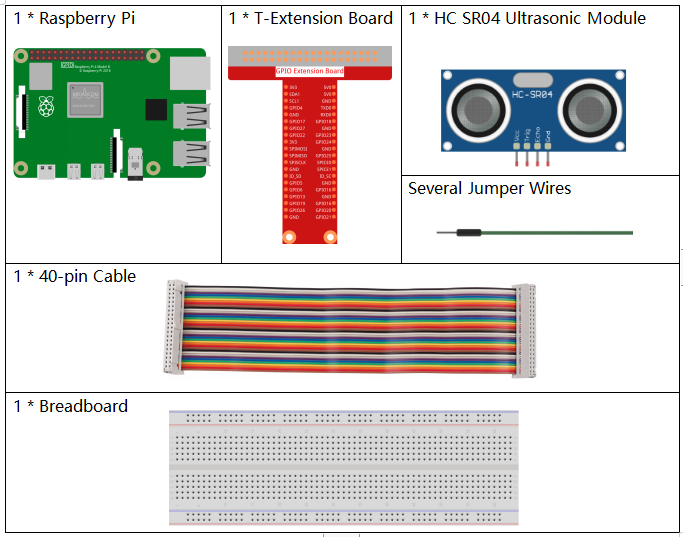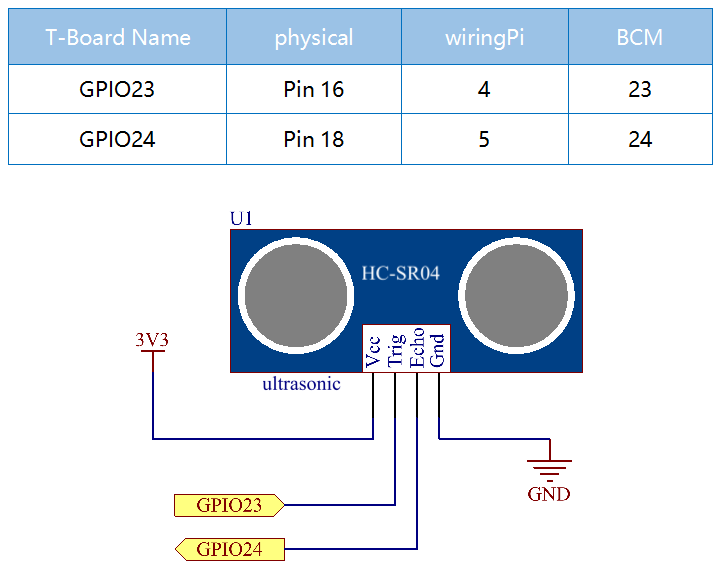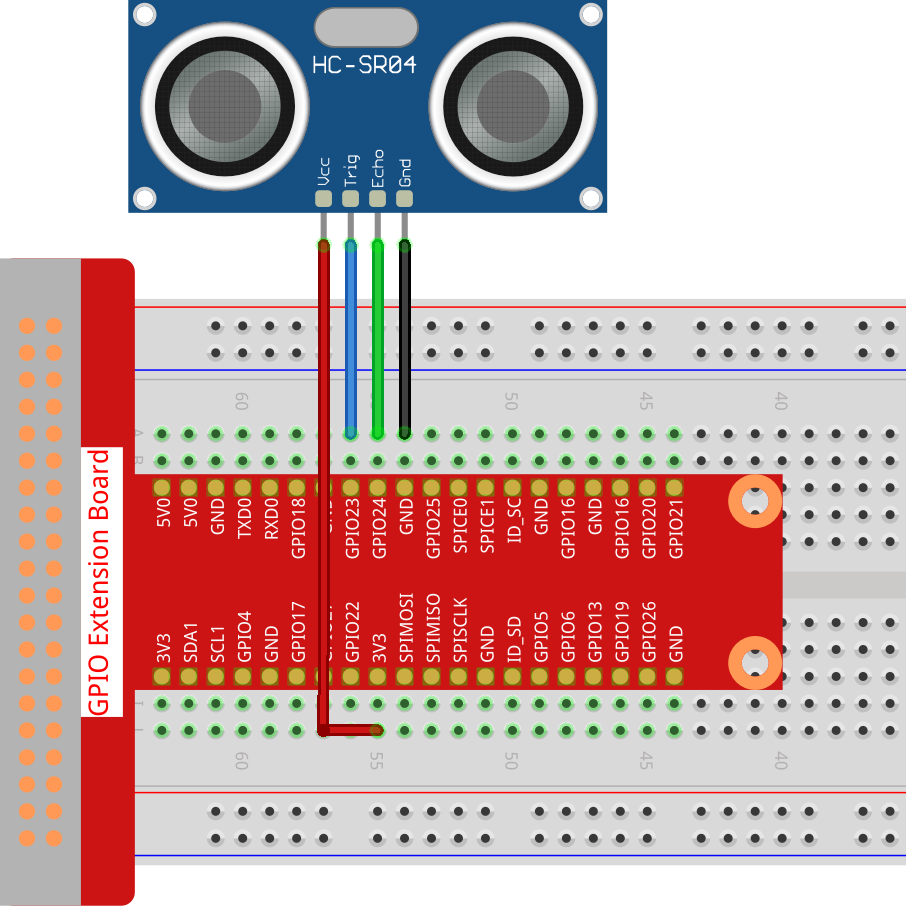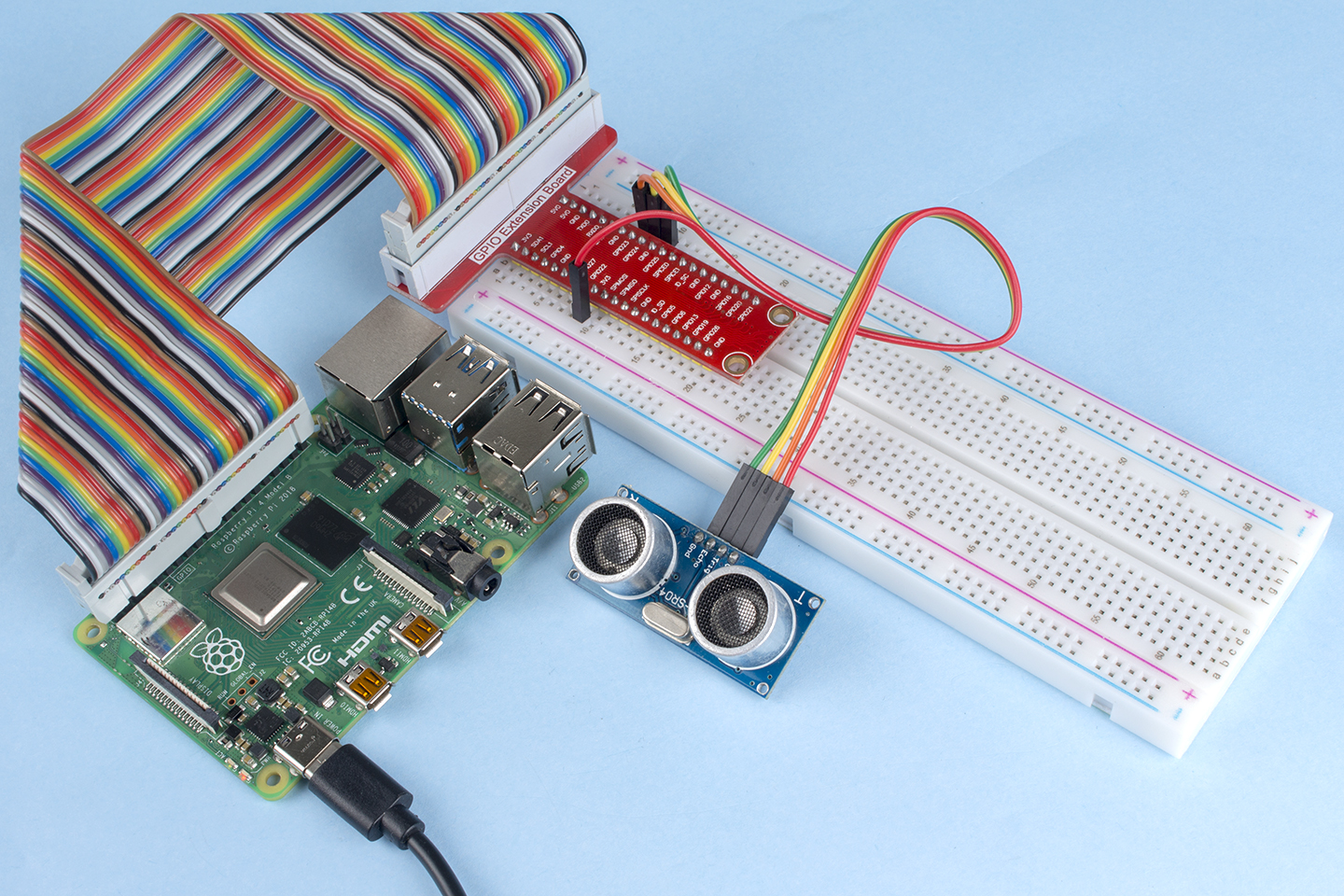# 2.2.8 Ultrasonic Sensor Module¶

## Introduction¶

The ultrasonic sensor uses ultrasonic to accurately detect objects and measure distances. It sends out ultrasonic waves and converts them into electronic signals.

## Components¶## Schematic Diagram¶## Experimental Procedures¶

Step 1: Build the circuit.Step 2: Go to the folder of the code.

```cd /home/pi/raphael-kit/python/
```

Step 3: Run the executable file.

```sudo python3 2.2.8_Ultrasonic.py
```

With the code run, the ultrasonic sensor module detects the distance between the obstacle ahead and the module itself, then the distance value will be printed on the screen.

Code

Note

You can Modify/Reset/Copy/Run/Stop the code below. But before that, you need to go to source code path like `raphael-kit/python`. After modifying the code, you can run it directly to see the effect.

```import RPi.GPIO as GPIO
import time

TRIG = 16
ECHO = 18

def setup():
GPIO.setmode(GPIO.BOARD)
GPIO.setup(TRIG, GPIO.OUT)
GPIO.setup(ECHO, GPIO.IN)

def distance():
GPIO.output(TRIG, 0)
time.sleep(0.000002)

GPIO.output(TRIG, 1)
time.sleep(0.00001)
GPIO.output(TRIG, 0)

while GPIO.input(ECHO) == 0:
a = 0
time1 = time.time()
while GPIO.input(ECHO) == 1:
a = 1
time2 = time.time()

during = time2 - time1
return during * 340 / 2 * 100

def loop():
while True:
dis = distance()
print ('Distance: %.2f' % dis )
time.sleep(0.3)

def destroy():
GPIO.cleanup()

if __name__ == "__main__":
setup()
try:
loop()
except KeyboardInterrupt:
destroy()
```

Code Explanation

```def distance():
```

This function is used to realize the function of ultrasonic sensor by calculating the return detection distance.

```GPIO.output(TRIG, 1)
time.sleep(0.00001)
GPIO.output(TRIG, 0)
```

This is sending out a 10us ultrasonic pulse.

```while GPIO.input(ECHO) == 0:
a = 0
time1 = time.time()
```

This empty loop is used to ensure that when the trigger signal is sent, there is no interfering echo signal and then get the current time.

```while GPIO.input(ECHO) == 1:
a = 1
time2 = time.time()
```

This empty loop is used to ensure that the next step is not performed until the echo signal is received and then get the current time.

```during = time2 - time1
```

Execute the interval calculation.

```return during * 340 / 2 * 100
```

The distance is calculated in the light of time interval and the speed of sound propagation. The speed of sound in the air: 340m/s.

## Phenomenon Picture¶